Note: Functions listed with a ✔ are custom functions

## Goal:

The goals of this notebook are to:

This notebook does not use DICOMS

For example: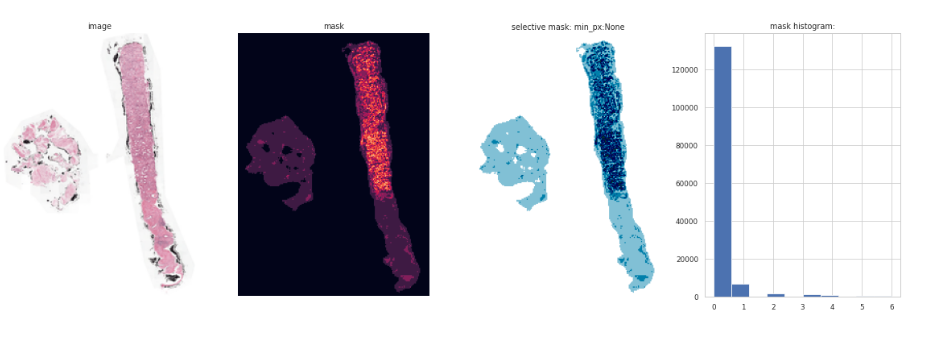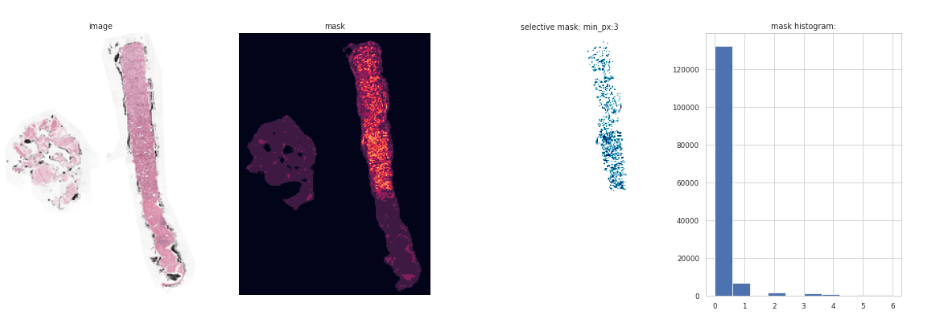## Motivation

There more than 1 million new prostate cancer diagnoses reported every year, in fact prostate cancer is the second most common cancer among males worldwide that results in more than 350,000 deaths annually. Diagnosis of prostate cancer is based on the grading of prostate tissue biopsies. These tissue samples are examined by a pathologist and scored according to the Gleason grading system.

The grading process consists of finding and classifying cancer tissue into so-called Gleason patterns (3, 4, or 5) based on the architectural growth patterns of the tumor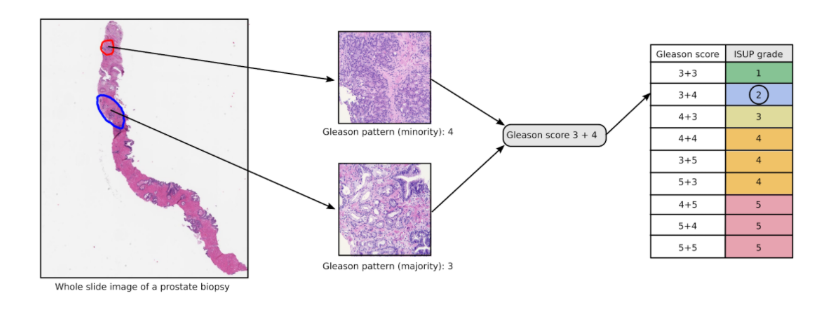(picture: courtesy: Kaggle)

After the biopsy is assigned a Gleason score, it is converted into an ISUP grade on a 1-5 scale

## Dataset

#Load the dependancies
from fastai.basics import *
from fastai.callback.all import *
from fastai.vision.all import *

import seaborn as sns
import numpy as np
import pandas as pd
import os
import cv2

sns.set(style="whitegrid")
sns.set_context("paper")

matplotlib.rcParams['image.cmap'] = 'ocean_r'

source = Path("D:/Datasets/prostate_png")
files = os.listdir(source)
train = source/'train_images'

def view_image(folder, fn):
if folder == train:
filename = f'{folder}/{fn}.png'
file = Image.open(filename)
t = tensor(file)
if folder == train:
show_image(t)
show_image(t[:,:,2])


Lets view an image

view_image(train, '0005f7aaab2800f6170c399693a96917')view_image(mask, '0005f7aaab2800f6170c399693a96917')The dataset is categorized by both isup_grade and gleason_score. What is noticed is that the masks have different intensities. For example we can specify a function that will display the image, the mask and plot a histogram of the intensites.

def view_images(file, mask, fn):
ima = f'{file}/{fn}.png'
ima_file = Image.open(ima); ima_t = tensor(ima_file)
ima_msk = Image.open(msk); msk_t = tensor(ima_msk)

fig, (ax1, ax2, ax3) = plt.subplots(1,3, figsize = (20, 6))
s1 = show_image(ima_t, ax=ax1, title='image')
plt.show()

view_images(train, mask, '06636cdd43041e78141f2f5069fa62d5')Plotting a histogram of the mask intensities shows that the bulk of the intensity is between 0 and 1 and this corresponds to the the bulk of the pixels which is the outline of the mask (light blue)

Here are some more examples

view_images(train, mask, '0d3159cd1b2495cc82637ececf63ed41')view_images(train, mask, '08134913a9aa1d541f719e9f356f9378')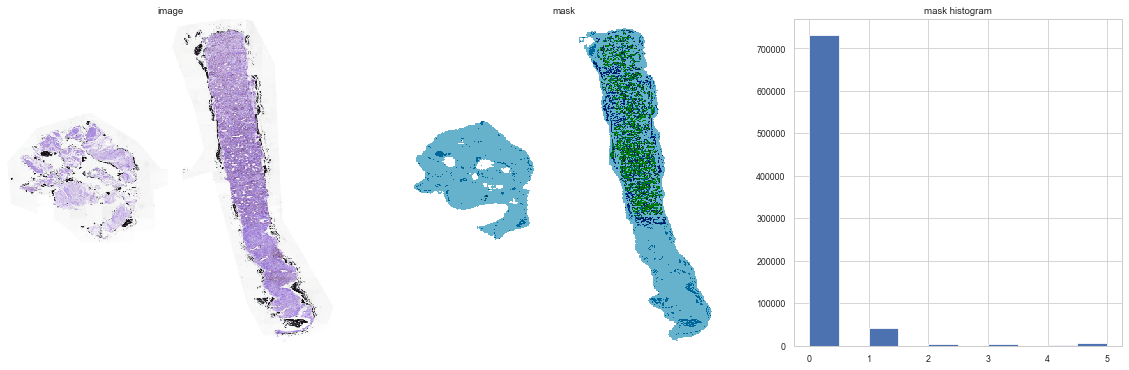Can see that the bulk of the pixels within the mask are the light blue areas of the mask which correspond to the the out outline of the mask itself.

To be able to view the mask images at different intensities I adapted a function from fastai's medical imaging library (which is typically geared towards working with DICOM images).

from fastai.medical.imaging import *


This library has a show function that has the capability of specifying max and min pixel values so you can specify the range of pixels you want to view within an image (useful when DICOM images can vary in pixel values between the range of -32768 to 32768).

You can easily adapt any function in fastai2 using @patch and it just works! In this case I am adapting the show function so you can specify min and max pixel values for this dataset.

@patch
@delegates(show_image)
def show(self:PILImage, scale=True, cmap=plt.cm.ocean_r, min_px=None, max_px=None, **kwargs):
px = tensor(self)
if min_px is not None: px[px<min_px] = float(min_px)
if max_px is not None: px[px>max_px] = float(max_px)
show_image(px, cmap=cmap, **kwargs)


We will also have to define another function that will allow us to view the selective masks

def selective_mask(file, mask, fn, min_px=None, max_px=None):
ima = f'{file}/{fn}.png'
ima_file = Image.open(ima); ima_t = tensor(ima_file)
ima_msk = Image.open(msk); msk_t = tensor(ima_msk)
msk_pil = PILImage.create(msk_t[:,:,2])

fig, (ax1, ax2, ax3, ax4) = plt.subplots(1,4, figsize = (20, 6))
s1 = show_image(ima_t, ax=ax1, title='image')
s3 = msk_pil.show(min_px=min_px, max_px=max_px, ax=ax3, title=f'selective mask: min_px:{min_px}')
plt.show()


The plot shows the original image, the mask, the selective mask(in this case all intensities are shown hence the reason it looks the same as the mask image) and the histogram of intensities (again the bulk of pixels are within 0 and 1

selective_mask(train, mask, '08134913a9aa1d541f719e9f356f9378', min_px=None, max_px=None)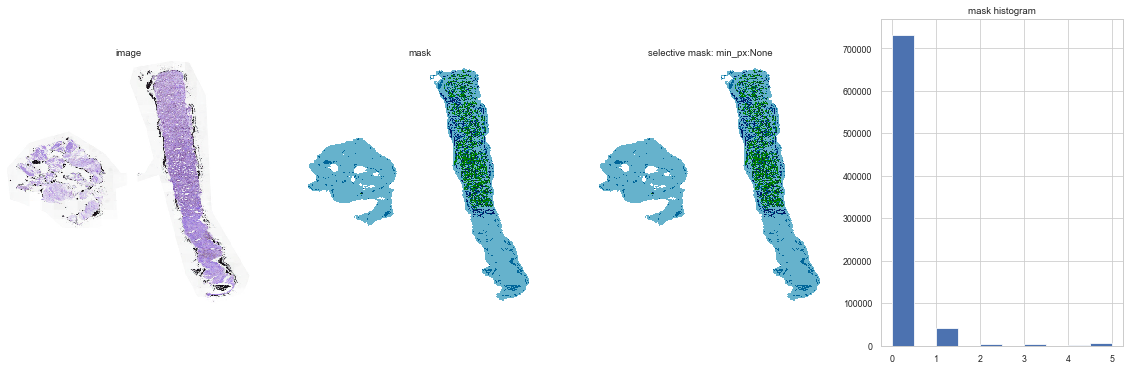How about intensities above 1 (so getting rid of the bulk of pixels)

selective_mask(train, mask, '08134913a9aa1d541f719e9f356f9378', min_px=1, max_px=None)Intensities above 2

selective_mask(train, mask, '08134913a9aa1d541f719e9f356f9378', min_px=2, max_px=None)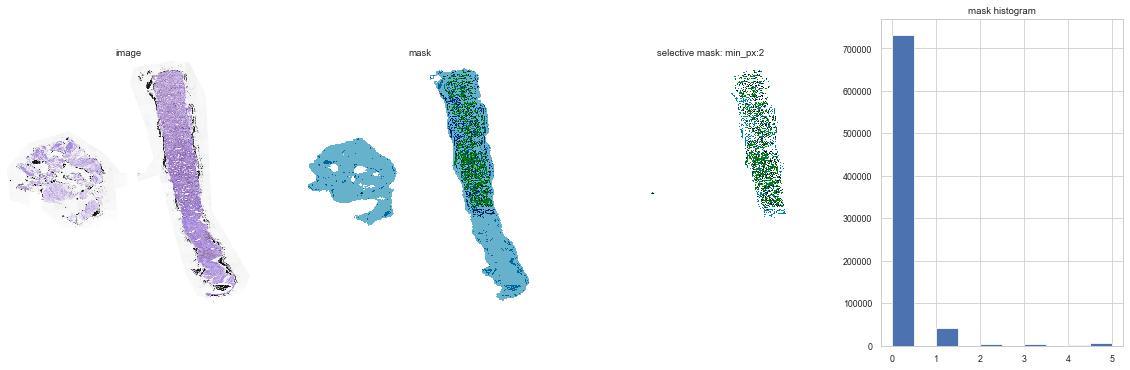Intensities above 3

selective_mask(train, mask, '08134913a9aa1d541f719e9f356f9378', min_px=3, max_px=None)The histogram does show some pixels above 4 but not many

selective_mask(train, mask, '08134913a9aa1d541f719e9f356f9378', min_px=4, max_px=None)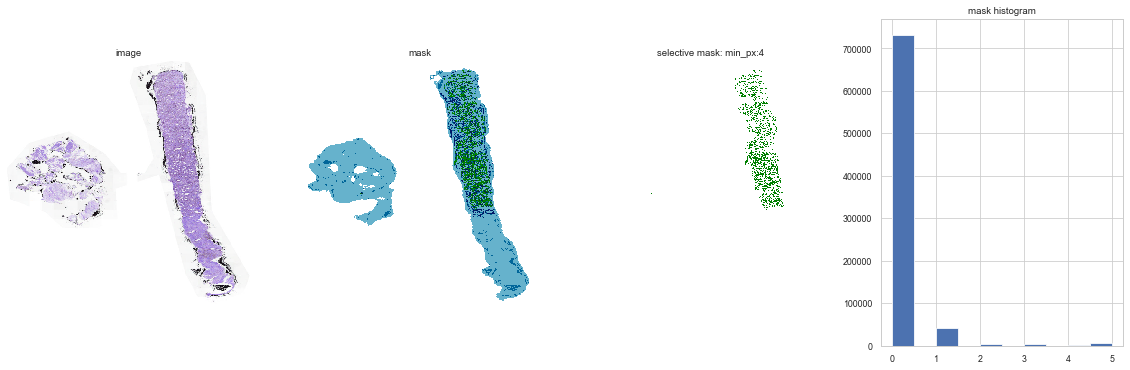Looking at the selective masks side by side

msk = f'{mask}/08134913a9aa1d541f719e9f356f9378_mask.png'
ima_msk = Image.open(msk); msk_t = tensor(ima_msk)
msk_pil = PILImage.create(msk_t[:,:,2])

fig, (ax1, ax2, ax3, ax4, ax5) = plt.subplots(1,5, figsize = (20, 6))
s1 = msk_pil.show(min_px=None, max_px=None, ax=ax1, title='original mask')
s2 = msk_pil.show(min_px=1, max_px=2, ax=ax2, title='1 and 2')
s3 = msk_pil.show(min_px=2, max_px=3, ax=ax3, title='2 and 3')
s4 = msk_pil.show(min_px=3, max_px=4, ax=ax4, title='3 and 4')
s4 = msk_pil.show(min_px=4, max_px=5, ax=ax5, title='4 and 5')
plt.show()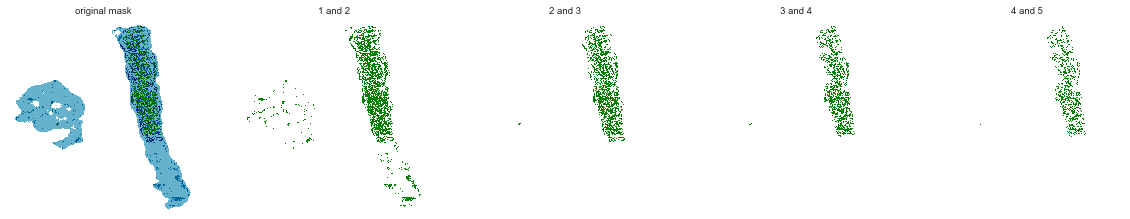isup_0 = train_labels[train_labels.isup_grade == 0]

selective_mask(train, mask, '0005f7aaab2800f6170c399693a96917', min_px=None, max_px=None)